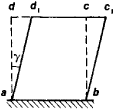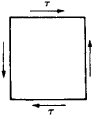# shear

(redirected from shear injury)
Also found in: Dictionary, Thesaurus, Medical.

## shear:

see strength of materialsstrength of materials,
measurement in engineering of the capacity of metal, wood, concrete, and other materials to withstand stress and strain. Stress is the internal force exerted by one part of an elastic body upon the adjoining part, and strain is the deformation or change in
.

## Shear

A shear of a plane relative to a line l in that plane is an affine transformation of the plane such that all points on / are fixed and all points on a line that is at unit distance from l undergo a shift by a vector k parallel to l; the points of a line that is at a distance ρ from l undergo a shift by the vector pk. A shear of space relative to a given plane is defined analogously.

## Shear

in strength of materials, a deformation of an elastic body wherein the applied forces cause a relative displacement of parallel layers or fibers of the material while the distance between the layers remains unchanged.Figure 1

An example of shear is the deformation of a right rectangular prism (Figure l) whose base ab is fixed and to whose upper face a shearing force is applied parallel to the base. The magnitude of the displacement cc1 = dd1 is the total shearing deformation. The angle γ is the shearing strain: because the deformations involved are small, γ ≈ tan γ = cc1/bc.Figure 2

In the case of pure shear, only the shearing stresses τ act along the faces of the prism (Figure 2). The equation τ = Gγ then holds, where G is the shearing modulus of elasticity, or shear modulus. Pure shear is a special case of plane strain and can therefore be investigated on the basis of one of the theories of strength (seeSTRENGTH OF SOLIDS). Tests of shearing strength are made, for example, for screw joints, riveted joints, welds, and notchings.

L. V. KASABTAN

## shear

[shir]
(design engineering)
A cutting tool having two opposing blades between which a material is cut.
(engineering)
An apparatus for hoisting heavy loads consisting of two or more poles fastened together at their upper ends and spread apart at their lower ends, secured or steadied by a guy or guys, and provided with a tackle. Also known as shear legs.
(mechanics)
(mining engineering)
To make vertical cuts in a coal seam that has been undercut.

## Shear

A straining action wherein applied forces produce a sliding or skewing type of deformation. A shearing force acts parallel to a plane as distinguished from tensile or compressive forces, which act normal to a plane. Examples of force systems producing shearing action are forces transmitted from one plate to another by a rivet that tend to shear the rivet, forces in a beam that tend to displace adjacent segments by transverse shear, and forces acting on the cross section of a bar that tend to twist it by torsional shear (see illustration). Shear forces are usually accompanied by normal forces produced by tension, thrust, or bending. Shearing stress is the intensity of distributed force expressed as force per unit area. See Stress and strain

## shear

1. A deformation (e.g., in a beam or flexural member) in which parallel planes slide relative to each other so as to remain parallel.
2. To cut a metal with a pair of moving blades or with one moving blade and one fixed edge.
3.See shears.

## shear

1. a form of deformation or fracture in which parallel planes in a body or assembly slide over one another
2. Physics the deformation of a body, part, etc., expressed as the lateral displacement between two points in parallel planes divided by the distance between the planes
3. a machine that cuts sheet material by passing a knife blade through it
4. a device for lifting heavy loads consisting of a tackle supported by a framework held steady by guy ropes
Site: Follow: Share:
Open / Close